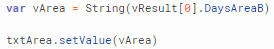# Javascript lookup with multiple criteria help

Hi, I've run into what I assume is an annoying syntax problem.

What I want it quite simple; to return a value from a query column based off two criteria. Similar to how you would use a dlookup in VBA.

The query in question has a transformer enabled to format the data as an array.
ie. return formatDataAsArray(data)

I've had success with a single criteria using the script below. I've place this in a click event on a button.

var vResult = QUERYNAME.data.find(x => x['ID'] == 2).COLUMNTORETURN

TEXTBOX.setValue(vResult)

This works and populated the textbox with the correct value.

In theory I would want something like this:

var vResult = QUERYNAME.data.find(x => x['COLUMN1'] == TEXTBOX1.value && x => x['COLUMN2'] == TEXTBOX2.value).COLUMNTORETURN

TEXTBOX.setValue(vResult)

However I'm missing something basic in the syntax or I'm using the incorrect function for multiple criteria.

Any help would be greatly appreciated

Hey @JoshuaWaters,

You want to filter by both conditions being true right?

``````var vResult = QUERYNAME.data.filter((x) => {
return x.column_1 == TEXTBOX1.value && x.column_2 == TEXTBOX2.value
})
``````

If you want to filter by at least one of the conditions being true change `&&` to `||`.

Does that work for you?

Hi again @minijohn

Yes both conditions being true, I should have stated that.

Thanks so much, I'll give it a try tomorrow and let you know.

No worries :)) let me know!

@minijohn Hi, it never worked sadly. For better context here's some screenshots. Below is the modal containing the form where my textboxes are and the button to run the script. I've also included the record the script should return. I have also made sure the query ran before running the script so the array of data should be ready.

I need a place to define which column to return.

Is `txtDay 18` a text component that parses the actual force date component?

I would use the filter values directly from the date comp.

``````var vResult  = QForceVsHarvestDates.filter((x) => {
return x.MonthPlanted == moment(actualForceDate.value).format('M') && x.DayPlanted == moment(actualForceDate.value).format('M')
})
``````

That should work, If it doesn't I would check the outputs of each variable we define in the filter and run the logic manually.

to return a column you can

``````vResult.DaysAreaA
``````

Does that help at all :))

1 Like

Yes that does help @minijohn thanks. The 2nd method doesn't work but the first does when I place the vResult.DaysAreaA in a variable like so:The main issue is it seems the query has to be pre-run for it to work and it seems like the code does not wait for QUERY.trigger() to complete before continuing on. Below is an example where this is tripping me up.Hey @JoshuaWaters, One way to set the value of vAreaResult only after the query has returned its data would be to pass a function (that sets the value of vAreaResult) to the query.trigger() function as the onSuccess optional parameter.

For example:

`QAreaB.trigger({`

`onSuccess: (data) => vAreaResult = data.DaysAreaB`

`})`

https://docs.retool.com/docs/scripting-retool#all-queries

Hopefully that helps for your use case!

\